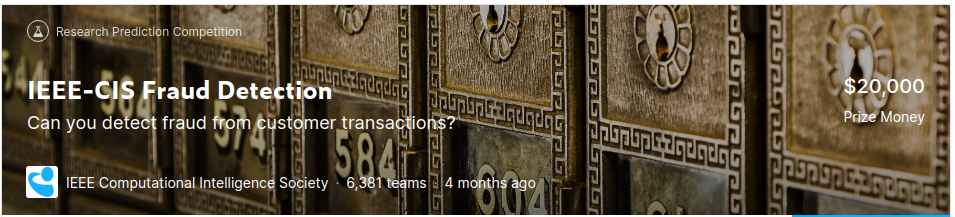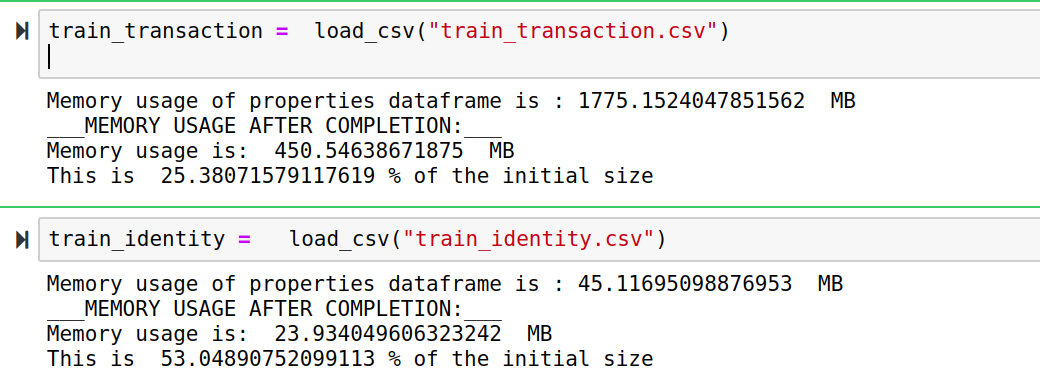# IntroductionAuthor: Ayush Chaurasia

In this tutorial, we’ll see how you can use W&B in a Kaggle competition. We'll also see how W&B's scikit-learn integration enables you to visualize performance metrics for your model with a single line of code. Finally, we'll run a hyperparameter sweep to pick the best model.

## Github repo →.

We'll be taking part in the IEE-CIS-Fraud Detection competition.

# The Dataset

## Let’s start by looking at the dataset.

Each row of the dataset consists of the details about a particular transaction, and whether the transaction is fraud or not. You can find a detailed exploratory data analysis in the accompanying repo, which will be helpful in understanding the datatset. For the sake of this report, we’ll stick to the model training and performance visualization.

The data is broken into two files `identity` and `transaction`, which are joined by `TransactionID`. Not all transactions have corresponding identity information. The `TransactionDT` feature is a timedelta from a given reference datetime (not an actual timestamp).

## Transaction

• ProductCD
• card1 - card6
• P_emaildomain
• R_emaildomain
• M1 - M9

## Identity

• DeviceType
• DeviceInfo
• id_12 - id_38

As always, we’ll use pandas to load the data, which is in the form of a .csv file.

But there’s a catch.

As the dataset is quite large and the numerical values are stored as float64, your Jupyter notebook might run out of memory and the kernel might die abruptly. To avoid this I’ve provided a helper function which tries to quantize the numerical values to reduce the memory used.

Here’s a link to the kaggle discussion that provided this solution for reducing memory usage. The helper function is called `load_csv` and it also provides the details about the memory usage.## Data Preprocessing

There are 434 columns in total, most of which are redundant and don’t contribute much to the learning. So, we’ ll get rid of all the useless data in the first step of pre-processing. These are the attributes that we use to train our model –

``````["TransactionID","DeviceType","DeviceInfo","TransactionDT",
"TransactionAmt","ProductCD","card4","card6","P_emaildomain",
``````

# Choosing The Model

As this is a classification problem, we’ll compare the performances of some of the most famous machine learning algorithms used for classification using Weights and Biases. Our experiment includes Logistic Regression, RandomForest and XGBoost classifiers.

Before we can log our model performance, we need to import the Weights & Biases library, which comes baked into Kaggle kernels.

``````import wandb
``````

Next, we’ll define a separate function for training and logging each classifier as it provides more flexibility in terms of logging as we can choose the metrics to be logged and the method used for each classifier. Here’s the code snippet for Logistic Regression.

``````def randomForestClassifier():
from sklearn.ensemble import RandomForestClassifier
clf = RandomForestClassifier(n_estimators=10)
clf.fit(X_train, y_train)
preds = clf.predict(X_test)
pred_prob = clf.predict_proba(X_test)
print(metrics.classification_report(y_test, preds))

# Log any metric with Weights and Biases
wandb.log({'accuracy_score': metrics.accuracy_score(y_test,preds)})
``````

Logging the performance metrics of scikit classifiers using Weights and Biases is simple. You can learn more about the different scikit plots supported by W&B here.

``````    # Learning curve
wandb.sklearn.plot_learning_curve(clf, X_train, y_train)
# Confusion Matrix
wandb.sklearn.plot_confusion_matrix(y_test, preds, clf.classes_)
# ROC Curve
wandb.sklearn.plot_roc(y_test, pred_prob, clf.classes_)
# Precision Recall Curve
wandb.sklearn.plot_precision_recall(y_test, pred_prob, clf.classes_)
``````

Here, I have logged all the metrics that can be using weigths and biases. This manual process of logging enables you to choose and log only the ones that you want to compare but if you want to log all of these metrics without making any customizations, then you can do so with a single line of code. So, let’s try that with out next classifier, Logistic Regression.

``````    def logisticRegressionClassifier():
from sklearn.linear_model import LogisticRegression
clf = LogisticRegression(solver='lbfgs', max_iter=4000)
clf.fit(X_train, y_train)
preds = clf.predict(X_test)
pred_prob = clf.predict_proba(X_test)
print(metrics.classification_report(y_test, preds))

wandb.log({'accuracy_score': metrics.accuracy_score(y_test,preds)})
wandb.sklearn.plot_classifier(clf, X_train, X_test, y_train, y_test, preds, pred_p     rob, clf.classes_,model_name='LogisticRegression', feature_names=None)
``````

Here we’ve manually logged just one metric, i.e, accuracy_score, because we’ll run a sweep to maximize accuracy later. All the other metrics and visualizations from the previous classifier are logged using the function `wandb.sklearn.plot_classifier`. This function takes all the necessary parameters for calculations of metrics that can be used to evaluate a classifier and logs them directly to the Weights and Biases dashboard.

Finally, let’s define our XGBoost classifier

``````    import xgboost as xgb
def xgbClassifier():
print('xgboost')
param={}
xg_train = xgb.DMatrix(X_train, label=y_train)
xg_test = xgb.DMatrix(X_test, label=y_test)
watchlist = [(xg_train, 'train'), (xg_test, 'test')]
param['objective'] = 'multi:softmax'
# scale weight of positive examples
param['eta'] = 0.1
param['num_class'] = 2
bst = xgb.train(param, xg_train, 5, watchlist, callbacks=[wandb.xgboost.wandb_call     back()])
preds = bst.predict(xg_test)
wandb.log({'accuracy_score': metrics.accuracy_score(y_test,preds)})
``````

This is how any traditional XGBoost classfier is trained and this approach is independent of scikit-learn library as we’ve used XGBoost’s own data types, i.e, DMatrix. The wandb library also supports native XGBoost integration and the training and performance of the classifier and be logged by using `wandb.xgboost.wandb_callback()` as the callback function in the trainer.

XGBoost also provides scikit compatible API. So it can also be logged using wandb just like other scikit classifiers.

``````        clf = xgb.XGBClassifier(nthread = -1)
clf.fit(X_train, y_train)
preds = clf.predict(X_test)
pred_prob=clf.predict_proba(X_test)

# Learning curve
wandb.sklearn.plot_learning_curve(clf, X_train, y_train)
# Confusion Matrix
wandb.sklearn.plot_confusion_matrix(y_test, preds, clf.classes_)
# ROC Curve
wandb.sklearn.plot_roc(y_test, pred_prob, clf.classes_)
# Precision Recall Curve
wandb.sklearn.plot_precision_recall(y_test, pred_prob, clf.classes_)
``````

# Setting Up A Hyperparameter Sweep

We can use a hyperparameter sweep to find the best model, along with the hyperparameters for that model. For an SVM, we might use this to find the bets values of `C`, `gamma` and `kernel` type.

Here's how we can find the parameters to sweep over, and the search strategy:

``````    sweep_config = {
'method': 'random', #grid, random
'metric': {
'name': 'accuracy_score',
'goal': 'maximize'
},
'parameters': {
'model':{
'values':['randomForest','logistic','xgboost']
}}}
sweep_id = wandb.sweep(sweep_config)
``````

We need to define a function with the model training code, which is then called by the sweep agent with different combinations of hyperparameters.

``````    def train():
wandb.init()
if wandb.config.model == 'logistic':
logisticRegressionClassifier()

if wandb.config.model == 'randomForest':
randomForestClassifier()
if wandb.config.model == 'xgboost':
xgbClassifier()
``````

The final step is to call the agent and run the sweep.

``````    wandb.agent(sweep_id,function=train)
``````

# Visualizing the Hyperparameter Sweep

## Calibration Curve

When performing classification one often wants to predict not only the class label, but also the associated probability. This probability gives some kind of confidence on the prediction.

As it can be seen from the graph, all our models almost overlap the perfectly calibrated curve, so we don’t need to perform any manual calibration on our models.

## Summary Metrics

If you want to analyze all the performance metrics of a model in one graph, you can use the summary metric chart which has all the performance metrics such as accuracy, F1 socre, precision and recall in form of histograms.

## Class Proportions

In theory, our classifiers might achieve high degrees of accuracy by predicting all transactions and not fraud.

Class proportion plots visualize the label proportions as well as the train and test set distribution. So, this chart shows that the classes in this dataset are highly disproportionate. This might be the reason why all the classifiers have achieved a very high accuracy score, and we should perhaps try up-sampling the minority class (fraud transactions), down-sampling the majority class or increase the cost of classification mistakes on the minority class.

## Precision Recall Curve

Precision and recall are among the most important metrics used to evaluate the performance of a model. Generally these are combined using specific formulas to generate new metrics such Average Precision(AP) and Mean Average Precision(mAP).

## ROC Curve

Receiver Operating Characteristic (ROC) is to evaluate classifier output quality.

ROC curves typically feature true positive rate on the Y axis, and false positive rate on the X axis. This means that the top left corner of the plot is the “ideal” point - a false positive rate of zero, and a true positive rate of one. This is not very realistic, but it does mean that a larger area under the curve (AUC) is usually better.

# Conclusion

In this report you’ve learned how to track the results of your experiments when you’re competing in an online competition. The top submissions to Kaggle competitions usually differ by decimal points, so choosing a model or a set of hyperparameters that even slightly improves the performance will give you a huge boost in the leaderboards. Weights and biases lets you automate that process with a beautifully designed API and easy to customize visualizations!

We hope you find them useful in your own Kaggle submissions. Good luck!